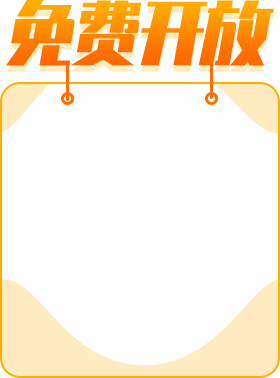# C++ 与C如何区分？

1、发展历史

1980年，Bjarne Stroustrup博士开始着手创建一种模拟语言，能够具有面向对象的程序设计特色。在当时，面向对象编程还是一个比较新的理念，Stroustrup博士并不是从头开始设计新语言，而是在C语言的基础上进行创建。这就是C++语言。

1985年，C++开始在外面慢慢流行。经过多年的发展，C++已经有了多个版本。为次，ANSI和ISO的联合委员会于1989年着手为C++制定标准。1994年2月，该委员会出版了第一份非正式草案，1998年正式推出了C++的国际标准。

2、C和C++

C++是C的超集，也可以说C是C++的子集，因为C先出现。按常理说，C++编译器能够编译任何C程序，但是C和C++还是有一些小差别。

例如C++增加了C不具有的关键字。这些关键字能作为函数和变量的标识符在C程序中使用，尽管C++包含了所有的C，但显然没有任何C++编译器能编译这样的C程序。

C程序员可以省略函数原型，而C++不可以，一个不带参数的C函数原型必须把void写出来。而C++可以使用空参数列表。

C++中new和delete是对内存分配的运算符，取代了C中的malloc和free。

标准C++中的字符串类取代了C标准C函数库头文件中的字符数组处理函数（C中没有字符串类型）。

C++中用来做控制态输入输出的iostream类库替代了标准C中的stdio函数库。

C++中的try/catch/throw异常处理机制取代了标准C中的setjmp()和longjmp()函数。

C++相对与C增加了一些关键字，如下：

typename bool dynamic_cast mutable namespace

static_cast using catch explicit new

virtual operator false private template

volatile const protected this wchar_t

const_cast public throw friend true

reinterpret_cast try

bitor xor_e and_eq compl or_eq

not_eq bitand

#include iostream.h

int main()

{

bool flag;

flag = true;

if(flag)

cout << true << endl;

return 0;

}

C++中还包括wchar_tt数据类型，wchar_t也是字符类型，但是是那些宽度超过8位的数据类型。许多外文字符集所含的数目超过256个，char字符类型无法完全囊括。wchar_t数据类型一般为16位。

#include iostream.h

int main()

{

wchar_t wc;

wc = 'b';

wout << wc;

wc = 'y';

wout << wc;

wc = 'e';

wout << wc << endl;

return 0;

}

有时候，根据表达式的需要，某个数据需要被当成另外的数据类型来处理，这时，就需要强制编译器把变量或常数由声明时的类型转换成需要的类型。为此，就要使用强制类型转换说明，格式如下：

int* iptr=(int*) &table;

long int el = 123;

short i = (int) el;

float m = 34.56;

int i = (int) m;

long int el = 123;

short i = int (el);

float m = 34.56;

int i = int (m);

在C语言中，输入输出是使用语句scanf()和printf()来实现的，而C++中是使用类来实现的。

#include iostream.h

main()    //C++中main()函数默认为int型，而C语言中默认为void型。

{

int a;

cout << input a number: ;

cin >> a;             /*输入一个数值*/

cout << a << endl;      //输出并回车换行

return 0;

}

cin,cout,endl对象，他们本身并不是C++语言的组成部分。虽然他们已经是ANSI标准C++中被定义，但是他们不是语言的内在组成部分。在C++中不提供内在的输入输出运算符，这与其他语言是不同的。输入和输出是通过C++类来实现的，cin和cout是这些类的实例，他们是在C++语言的外部实现。

在C++语言中，有了一种新的注释方法，就是‘//’，在该行//后的所有说明都被编译器认为是注释，这种注释不能换行。C++中仍然保留了传统C语言的注释风格/*……*/。

C++也可采用格式化输出的方法：

#include iostream.h

int main()

{

int a;

cout << input a number: ;

cin >> a;

cout << dec << a << ' '     //输出十进制数

<< oct << a << ' '     //输出八进制数

<< hex << a << endl;   //输出十六进制数

return 0;

}

1、无名的函数形参

声明函数时可以包含一个或多个用不到的形式参数。这种情况多出现在用一个通用的函数指针调用多个函数的场合，其中有些函数不需要函数指针声明中的所有参数。看下面的例子：

int fun(int x,int y)

{

return x*2;

}

尽管这样的用法是正确的，但大多数C和C++的编译器都会给出一个警告，说参数y在程序中没有被用到。为了避免这样的警告，C++允许声明一个无名形参，以告诉编译器存在该参数，且调用者需要为其传递一个实际参数，但是函数不会用到这个参数。下面给出使用了无名参数的C++函数代码：

int fun(int x,int) //注意不同点

{

return x*2;

}

2、函数的默认参数

C++函数的原型中可以声明一个或多个带有默认值的参数。如果调用函数时，省略了相应的实际参数，那么编译器就会把默认值作为实际参数。可以这样来声明具有默认参数的C++函数原型：

#include iostream.h

void show(int = 1,float = 2.3,long = 6);

int main()

{

show();

show(2);

show(4,5.6);

show(8,12.34,50L);

return 0;

}

void show(int first,float second,long third)

{

cout << first =<< first

<< second =<< second

<< third =<< third << endl;

}

1、参数个数不同

#include iostream.h

void a(int,int);

void a(int);

int main()

{

a(5);

a(6,7);

return 0;

}

void a(int i)

{

cout << i << endl;  //输出5

}

void a(int i,int j)

{

cout << i << j << endl;       //输出67

}

2.参数格式不同

#include iostream.h

void a(int,int);

void a(int,float);

int main()

{

a(5,6);

a(6,7.0);

return 0;

}

void a(int i,int j)

{

cout << i << j <<endl;          //输出56

}

void a(int i,float j)

{

cout << i << j << endl;          //输出67.0

}

C++语言中，允许变量定义语句在程序中的任何地方，只要在是使用它之前就可以；而C语言中，必须要在函数开头部分。而且C++允许重复定义变量，C语言也是做不到这一点的。看下面的程序：

#include iostream.h

int a;

int main()

{

cin >> a;

for(int i = 1;i <= 10; i++) //C语言中，不允许在这里定义变量

{

static int a = 0; //C语言中，同一函数块，不允许有同名变量

a += i;

cout<<::a<< <<a<<endl;

}

return 0;

}

1.为固定大小的数组分配内存

#include iostream.h

int main()

{

int *birthday = new int;

birthday = 6;

birthday = 24;

birthday = 1940;

cout << I was born on

<< birthday << '/' << birthday << '/' << birthday << endl;

delete [] birthday;      //注意这儿

return 0;

}

2.为动态数组分配内存

#include iostream.h

#include stdlib.h

int main()

{

int size;

cin >> size;

int *array = new int[size];

for(int i = 0;i < size;i++)

array = rand();

for(i = 0;i < size;i++)

cout << '\n' << array;

delete [] array;

return 0;

}

1.引用是一个别名

C++中的引用是其他变量的别名。声明一个引用型变量，需要给他一个初始化值，在变量的生存周期内，该值不会改变。& 运算符定义了一个引用型变量：

int a;

int& b=a;

#include iostream.h

int main()

{

int a = 123;

int& b = a;

cout << a << ','<< b << endl;       //输出123,123

a++;

cout << a << ','<< b << endl;       //输出124,124

b++;

cout << a<< ',' << b << endl;        //输出125,125

return 0;

}

2.引用的初始化

(1) 引用变量被声明为外部的，它可以在任何地方初始化

(2) 引用变量作为类的成员，在构造函数里对它进行初始化

(3) 引用变量作为函数声明的形参，在函数调用时，用调用者的实参来进行初始化

3.作为函数形参的引用

#include iostream.h

void func1(s p);

void func2(s& p);

struct s

{

int n;

char text;

};

int main()

{

static s str = {123,China};

func1(str);

func2(str);

return 0;

}

void func1(s p)

{

cout << p.n << endl;

cout << p.text << endl;

}

void func2(s& p)

{

cout << p.n << endl;

cout << p.text << endl;

}

4.以引用方式调用

#include iostream.h

void display(const Date&,const char*);

void swapper(Date&,Date&);

struct Date

{

int month,day,year;

};

int main()

{

static Date now={2,23,90};

static Date then={9,10,60};

display(now,Now: );

display(then,Then: );

swapper(now,then);

display(now,Now: );

display(then,Then: );

return 0;

}

void swapper(Date& dt1,Date& dt2)

{

Date save;

save=dt1;

dt1=dt2;

dt2=save;

}

void display(const Date& dt,const char *s)

{

cout << s;

cout << dt.month << '/' << dt.day << '/'<< dt.year << endl;

}

5.以引用作为返回值

#include iostream.h

struct Date

{

int month,day,year;

};

Date birthdays[]=

{

{12,12,60};

{10,25,85};

{5,20,73};

};

const Date& getdate(int n)

{

return birthdays[n-1];

}

int main()

{

int dt=1;

while(dt!=0)

{

cout<<Enter date # (1-3,0 to quit)<<endl;

cin>>dt;

if(dt>0 && dt<4)

{

const Date& bd = getdate(dt);

cout << bd.month << '/' << bd.day << '/'<< bd.year << endl;

}

}

return 0;

}

## 相关阅读